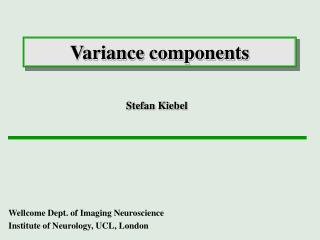DownloadDownload PresentationVariance components

# Variance components

Télécharger la présentation## Variance components

- - - - - - - - - - - - - - - - - - - - - - - - - - - E N D - - - - - - - - - - - - - - - - - - - - - - - - - - -
##### Presentation Transcript

1. Variance components Stefan Kiebel Wellcome Dept. of Imaging Neuroscience Institute of Neurology, UCL, London

2. Modelling in SPM functional data design matrix hypotheses smoothed normalised data parameter estimation general linear model pre-processing SPMs adjusted P-values templates variance components

3. general linear model error e normally distributed = + • model specified by • design matrix X • assumptions about e N: number of observations p: number of regressors

4. Summary Sphericity/non-sphericity Restricted Maximum Likelihood (ReML) Estimation in SPM2

5. Summary Sphericity/non-sphericity Sphericity/non-sphericity Restricted Maximum Likelihood (ReML) Estimation in SPM2

6. ‚sphericity‘ Scans ‚sphericity‘ means: i.e. Scans

7. ‚non-sphericity‘ non-sphericity means that the error covariance doesn‘t look like this*: *: or can be brought through a linear transform to this form

8. Example: serial correlations with autoregressive process of order 1 (AR(1)) autocovariance- function

9. Summary Sphericity/non-sphericity Restricted Maximum Likelihood (ReML) Estimation in SPM2

10. Restricted Maximum Likelihood observed ReML estimated

11. t-statistic (OLS estimator) c = +1 0 0 0 0 0 0 0 0 0 0 approximate degrees of freedom following Satterthwaite ReML-estimate

12. Variance components Variance components Q model the error The variance parameters l are estimated by ReML. model for inhomogeneous variances (2 groups) model for sphericity

13. Example I Auditory Presentation (SOA = 4 secs) of (i) words and (ii) words spoken backwards Stimuli: e.g. “Book” and “Koob” (i) 12 control subjects (ii) 11 blind subjects Subjects: Scanning: fMRI, 250 scans per subject, block design U. Noppeney et al.

14. Population differences Controls Blinds 1st level: 2nd level:

15. Summary Sphericity/non-sphericity Restricted Maximum Likelihood (ReML) Estimation in SPM2

16. Estimating variances EM-algorithm E-step M-step Assume, at voxel j: K. Friston et al. 2002, Neuroimage

17. voxelwise model specification parameter estimation Time hypothesis statistic Time Intensity Time series in one voxel SPM

18. Spatial ‚Pooling‘ • Assumptions in SPM2: • global correlation matrix V • local variance estimated observed ReML local in voxel j: global

19. Estimation in SPM2 ReML (pooled estimate) Ordinary least-squares ‚quasi‘-Maximum Likelihood • optional in SPM2 • one pass through data • statistic using (approximated) effective degrees of freedom • 2 passes (first pass for selection of voxels) • more precise estimate of V

20. t-statistic (ML-estimate) c = +1 0 0 0 0 0 0 0 0 0 0 ReML-estimate

21. Example II Stimuli: Auditory Presentation (SOA = 4 secs) of words Subjects: (i) 12 control subjects fMRI, 250 scans per subject, block design Scanning: What regions are affected by the semantic content of the words? Question: U. Noppeney et al.

22. Repeated measures Anova 1st level: Motion Sound Visual Action ? = ? = ? = 2nd level: